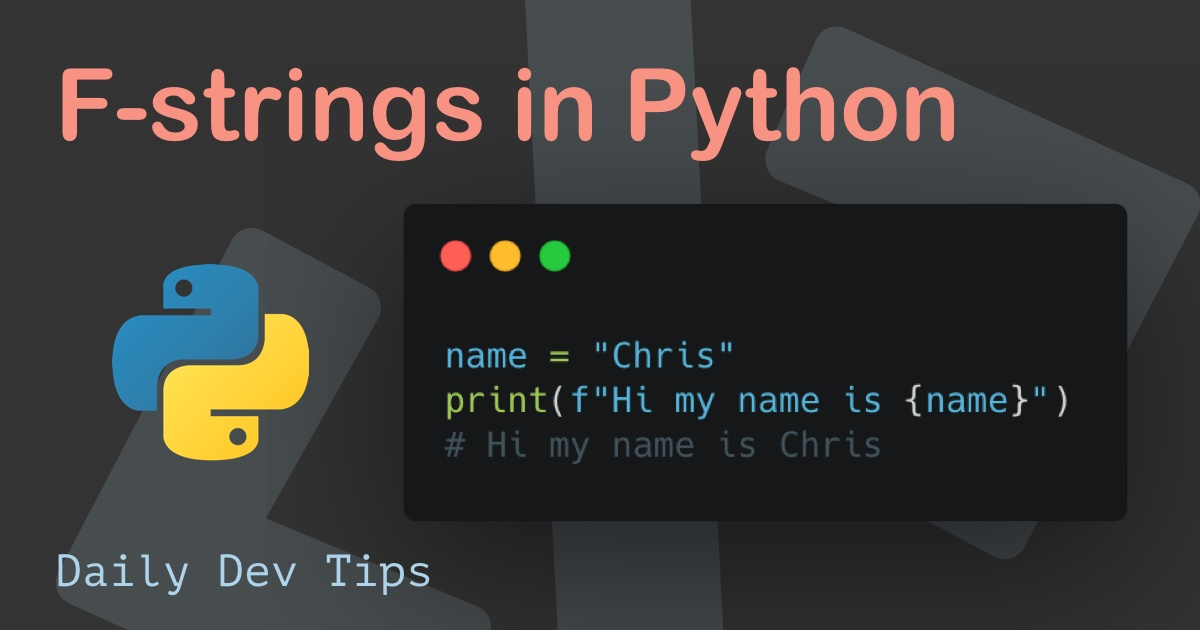Subscribe

# Python map() function

••••••• + 3 devs liked it

✍️

How to use the map() function in Python

5 Jun, 2021 · 2 min read

After looking into the Python filter function, let's take a look at how the map works.

As we learned, the filter will return a section of the input based on certain criteria.

## Map() function in Python permalink

Let's first have a look at the syntax:

``result = map(myFunction, input)``

To give more details to this:

• `result`: Is the output. This will be a changed sequence.
• `filter`: Is the Python built-in function
• `myFunction`: This will be a custom function we are going to build
• `input`: This is the original sequence we want to map

As you can see, the syntax looks like the filter function. The main change will be inside the `myFunction`.

Let's say we have a list of numbers that we need to multiply by themselves.

``````input = [2, 5, 10]

def myFunction(n):
return n * n

result = map(myFunction, input)
print(list(result))

# [4, 25, 100]``````

Pretty cool right, and like the filter one, we can use Lambda functions to make it even shorter.

``````input = [2, 5, 10]

result = map(lambda n: n * n, input)
print(list(result))

# [4, 25, 100]``````

Tweet this tip
••••••• + 3 devs liked it

### Speedtest your connection in Python

21 Jun, 2021 · 2 min read### F-strings in Python

7 Jun, 2021 · 2 min readJoin 2103 devs and subscribe to my newsletter

• 1000 articles written
• 2103 devs subscribed
• 529116 words written